Thursday, February 24, 2011

Support vector machines (SVMs) in F# using Microsoft Solver Foundation

Support vector machines are a super star in machine learning and data mining in the past decade. It reheats statistical learning in machine learning community. It is also one of the classifiers that work practically with applications to other disciplines such as bioinformatics.

In this post, I will give a brief review of the theory of this classifier and show an F# implementation using the quadratic programming (QP) solver in Microsoft Solver Foundation (MSF).

It may seem dull to start with mathematics. But the math in SVMs is actually quite intuitive and would be quite easy if one has some training in linear algebra and calculus. Also understanding the intuition behind the formulae is more important in the formulation itself. There are quite a lot of good SVM tutorials written by researchers. Thus I only write the important formulas that occur in the implementation. The reader could link the F# code with formulas directly.

My note is based on the machine learning course by Prof. Jaakkola [Jaakkola] and the Matlab implementation therein. The course material is highly comprehensive yet accurate.

Background review for SVMs

Given a binary labeled dataset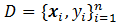, whereandis 1 or -1. Think about the data points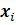plotted in a d dimensional (Euclidean) space, the linear SVM classifier is a hyperplane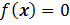in the space and it “best” separates the two kinds of data points. Here “best” means that the hyperplane should have the largest margin, which is the distance from the plane to the sides of labeled points. Intuitively the larger the margin is, the more robust and confident the classifier is. As if the hyperplane shakes a little, it still classifies well for its being far from both sides.

Let’s take d=2 as the discussing example: data points are plotted in a 2-D plane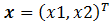. The aim is to draw a line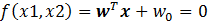to separate the two kinds of points such that the margin of the separation is maximized. In the following Figure, the line with maximum margin is shown. After some geometry work, the margin is calculated as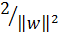, thus minimizing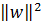with the constraints that the two kinds of points are well separated (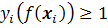) gives the max-margin hyperplane.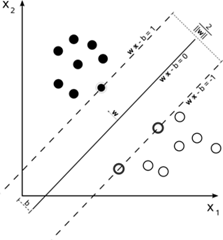(Wikipedia picture)

Two important extensions to the above simplest SVM are:

1. Allowing imperfect separation, that is when a hyperplane could not separate the points perfectly, it is allowed to mis-separate some data points. This introduces a slack variable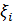for each data point, when the point is on the correct side of the plane, the slack variable is 0, otherwise it is measures the distance it goes from the plane minus 1, if the values goes to below zero set it zero. (Please read the Note and reference section for more explanation.)

2. Kernel. The simplest SVM is a linear classifier in the original space. However, there are situations where linear classifier is not sufficient (even the best), one strategy is to do a non-linear transformation or mapping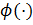that maps a data point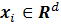to another space, so that a linear classifier in that space, which is non-linear in the original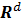space, does a better separation of the data points. The kernel trick is that we don’t need to defineexplicitly; only the definition of inner product of that space is required: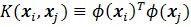.

With the two extensions, the new maximum margin objective becomes: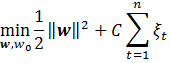subject to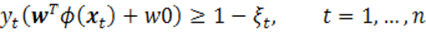The dual form: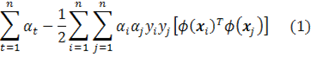subject to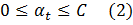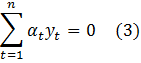The data points with its alpha value greater than 0 are called support vectors.

With the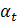in the dual problem solved, the SVM classification hyperplane is recovered by: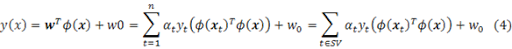The threshold parameter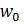could be calculated by substituting back to the support vectors: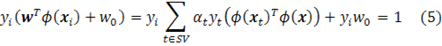Any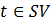and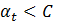(So that make sure data pointis on the margin boundary, not in-between) would give a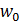, to stabilize the solution, it is common practice to take the median of off the possible.

The implementation

The solver is actually an Interior Point Solver, which could solve linear and quadratic programming with linear constraints.

Page 14-17 of MSF-SolverProgrammingPrimer.pdf show an example usage of the QP solver in Microsoft Solver Foundation. But the example is not complete and it does not demonstrate one very subtle part: how to set the coefficients for the quadratic terms.

The following code example shows a simple example with the the comment regarding the coefficient setting:

Example usage of the QP solver
1. #r @"C:\Program Files (x86)\Sho 2.0 for .NET 4\packages\Optimizer\Microsoft.Solver.Foundation.dll"
2. open Microsoft.SolverFoundation.Common
3. open Microsoft.SolverFoundation.Solvers
4. open Microsoft.SolverFoundation.Services
5. // test QP
6. module TEST =
7. (* minimize x^2 + y^2 + 3xy + 2x + y *)
8. (* notice that in the InteriorPointSolver,
9. the coefficients for xy & yx should be the same (so only set ONCE!)
10. if we set both 3xy and 0yx, the solver takes the later coef.
11. *)
12. let solver = new InteriorPointSolver()
13. let _, goal = solver.AddRow("dual objective value")
15. let _, x = solver.AddVariable("x")
16. let _, y = solver.AddVariable("y")
17. solver.SetCoefficient(goal, x, Rational.op_Implicit(2))
18. solver.SetCoefficient(goal, y, Rational.op_Implicit(1))
19. // for terms like x-y (where x != y), set its coef for only one time!
20. solver.SetCoefficient(goal, Rational.op_Implicit(3), x, y)
21. //solver.SetCoefficient(goal, Rational.Zero, y, x)
22. solver.SetCoefficient(goal, Rational.op_Implicit(1), x, x)
23. solver.SetCoefficient(goal, Rational.op_Implicit(1), y, y)
24. let param = new InteriorPointSolverParams()
25. solver.Solve(param) |> ignore
26. //solver.Result
27. let objectiveValue = solver.GetValue(0).ToDouble()
28. let x0 = solver.GetValue(1).ToDouble()
29. let y0 = solver.GetValue(2).ToDouble()
30. x0*x0 + y0*y0 + 3.0 * x0 * y0 + 2. * x0 + y0
31. //x0*x0 + y0*y0 + 0.0 * x0 * y0 + 2. * x0 + y0

The implementation of SVM is a straightforward translation of equations (1) to (5). The following shows the svm learning (bulidSvm) and testing (svmClassify) functions:

SVM Implementation
1. open System
2. type dataset =
3. { features: float array array; // (instance = float array) array
4. mutable labels: int array; //
5. }
6. with
7. member x.NumSamples = x.features.Length
8. module Array =
9. let median (a:'a array) =
10. let sorted = Array.sort a
11. sorted.[sorted.Length / 2]
12. module Kernel =
13. let linear a b =
14. Array.fold2 (fun acc p q -> acc + p * q) 0.0 a b
15. let polynomial k a b =
16. let dot = linear a b
17. Math.Pow(1.0 + dot, k |> float)
18. let gaussian beta a b =
19. let diff = Array.fold2 (fun acc p q -> acc + (p-q)*(p-q)) 0.0 a b
20. exp (-0.5 * beta * diff)
21. module SVM =
22. type svmmodel = {
23. SVs:dataset;
24. alpha:float array;
25. kernel: float[] -> float[] -> float;
26. w0:float;
27. }
28. with
29. member x.NumSupporVectors = x.SVs.features.Length
30. let buildSVM (ds:dataset) (C:float) (kernel:float[] -> float[] -> float) =
31. let n = ds.features.Length
32. let C = Rational.op_Implicit(C)
33. let zero = Rational.Zero
34. // create a interior point solver, which solves the QP problem
35. let solver = new InteriorPointSolver()
36. // set the objective value / goal
37. let _, goal = solver.AddRow("dual objective value")
38. // false == maximizing the objective value
39. // the value of goal is (1)
40. solver.AddGoal(goal, 0, false) |> ignore
41. // add the Lagangian variables \alpha_i and set their bounds (0 <= \alpha_i <= C)
42. let alpha = Array.create n 0
43. for i=0 to n-1 do
44. let _, out = solver.AddVariable("alpha_"+i.ToString())
45. alpha.[i] <- out
46. solver.SetBounds(out, zero, C)
47. // add contraint: \sum_i \alpha_i * y_i = 0
48. // equation (2)
49. let _, sumConstraint = solver.AddRow("SumConstraint")
50. solver.SetBounds(sumConstraint, zero, zero);
51. for i=0 to n-1 do
52. // set the coefs for the sum constraint
53. // equation (2)
54. solver.SetCoefficient(sumConstraint, alpha.[i], Rational.op_Implicit(ds.labels.[i]))
55. // add the \alpha_i terms into the objective
56. solver.SetCoefficient(goal, alpha.[i], Rational.One)
57. // add the qudratic terms
58. for j=0 to i do
59. // coef = y_i * y_j * K(x_i, x_j)
60. let coef = float(ds.labels.[i] * ds.labels.[j]) * (kernel ds.features.[i] ds.features.[j])
61. if i=j then
62. solver.SetCoefficient(goal, Rational.op_Implicit(-0.5 * coef), alpha.[i], alpha.[j])
63. else
64. solver.SetCoefficient(goal, Rational.op_Implicit(-coef), alpha.[i], alpha.[j])
65. // use the default parameters
66. let param = new InteriorPointSolverParams()
67. solver.Solve(param) |> ignore
68. // get the alpha values out
69. let alphaValue = Array.init n (fun i -> solver.GetValue(i+1))
70. (* print optimization result
71. printfn "goal value = %A" (solver.GetValue(0).ToDouble())
72. for i=1 to n do
73. printfn "%A" (solver.GetValue(i).ToDouble())
74. *)
75. let alphaNew = new ResizeArray<Rational>()
76. // extract the non-zero alpha values out and their corresponding support vectors
77. let SVs =
78. let feats = new ResizeArray<float[]>()
79. let labs = new ResizeArray<int>()
80. let maxAlpha = Array.max alphaValue
81. let threshold = maxAlpha * Rational.op_Implicit(1e-8)
82. for i=0 to n-1 do
83. if alphaValue.[i] > threshold then
87. { features = feats |> Seq.toArray;
88. labels = labs |> Seq.toArray;
89. }
90. // solve w_0 in the primal form
91. let alphaNZ = alphaNew |> Seq.toArray
92. // equation (5)
93. let w0 =
94. alphaNZ
95. |> Array.mapi (fun i a ->
96. if a = C then
97. None
98. else
99. let mutable tmp = 0.0
100. for j=0 to SVs.NumSamples-1 do
101. tmp <- tmp + alphaNZ.[j].ToDouble() * (SVs.labels.[j] |> float) * (kernel SVs.features.[i] SVs.features.[j])
102. Some ((float SVs.labels.[i]) - tmp)
103. )
104. |> Array.filter (fun v -> match v with None -> false | _ -> true)
105. |> Array.map (fun v -> match v with Some v -> v | _ -> 0.0)
106. |> Array.median
107. // construct an svm record
108. {
109. SVs = SVs;
110. alpha = alphaNZ |> Array.map (fun v -> v.ToDouble());
111. kernel = kernel;
112. w0 = w0;
113. }
114. let svmClassify (model:svmmodel) (ds:dataset) =
115. // equation (4)
116. let vals =
117. ds.features
118. |> Array.map (fun x ->
119. let mutable sum = 0.0
120. for i=0 to model.NumSupporVectors-1 do
121. sum <- sum + model.alpha.[i] * (float model.SVs.labels.[i]) * (model.kernel model.SVs.features.[i] x)
122. sum + model.w0
123. )
124. let nCorrect =
125. Array.map2 (fun value label -> if (value > 0.0) && (label = 1) || (value < 0.0) && (label = -1) then 1 else 0) vals ds.labels
126. |> Array.sum
127. (float nCorrect) / (float ds.NumSamples), vals

Try on the iris data set we used in the Logistic Regression post:

let svm = buildSVM iris 10.0 Kernel.linear
let classifyResult = svmClassify svm iris

val svm : svm =
{SVs =
{features =
[|[|1.0; 6.2; 2.2; 4.5; 1.5|]; [|1.0; 5.9; 3.2; 4.8; 1.8|];
[|1.0; 6.3; 2.5; 4.9; 1.5|]; [|1.0; 6.7; 3.0; 5.0; 1.7|];
[|1.0; 6.0; 2.7; 5.1; 1.6|]; [|1.0; 5.4; 3.0; 4.5; 1.5|];
[|1.0; 4.9; 2.5; 4.5; 1.7|]; [|1.0; 6.0; 2.2; 5.0; 1.5|];
[|1.0; 6.3; 2.7; 4.9; 1.8|]; [|1.0; 6.2; 2.8; 4.8; 1.8|];
[|1.0; 6.1; 3.0; 4.9; 1.8|]; [|1.0; 6.3; 2.8; 5.1; 1.5|];
[|1.0; 6.0; 3.0; 4.8; 1.8|]|];
labels = [|-1; -1; -1; -1; -1; -1; 1; 1; 1; 1; 1; 1; 1|];};
alpha =
[|6.72796421; 10.0; 10.0; 10.0; 10.0; 6.475497298; 1.490719712;
8.547262902; 3.165478894; 10.0; 10.0; 10.0; 10.0|];
kernel = <fun:svm@226-32>;
w0 = -13.63716815;}

>
Real: 00:00:00.002, CPU: 00:00:00.000, GC gen0: 0, gen1: 0, gen2: 0

val classifyResult : float * float [] =
(0.97,
[|-2.787610618; -2.380530973; -1.42477876; -2.929203541; -1.681415929;
-1.96460177; -1.24778761; -6.106194692; -2.761061946; -2.973451328;
…. 4.203539824; 1.82300885|])

Notes and references

The optimization in SVMs could be treated as a general QP problem as shown in our implementation. However, when the number of the data points is big, the QP grows too big to handle by the QP solver.

As a special QP problem, SVM has a lot of novel solutions proposed in the machine learning research area, e.g. the SMO approach [Platt] in LibSVM implementation and the Ball Vector Machine approach [Tsang] transforming the special QP into a (discrete) computational geometry problem, minimum enclosing ball. For practical usage of SVM, one usually uses these dedicated approaches.

Besides the maximum margin interpretation, SVMs also have theory roots in functional regularization theory, in which the optimizationis viewed as minimizing the error term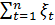while controlling the complexity of the function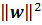and C is a tradeoff parameter between these two. The reader is referred to the teaching notes and papers by Prof. Poggio [Poggio].

[Burges] A Tutorial on Support Vector Machines for Pattern Recognition. http://research.microsoft.com/en-us/um/people/cburges/papers/svmtutorial.pdf

[Jaakkola] http://courses.csail.mit.edu/6.867/.

[Platt] Sequential Minimal Optimization: A Fast Algorithm for Training Support Vector Machines. ftp://ftp.research.microsoft.com/pub/tr/tr-98-14.pdf

[Poggio] http://www.mit.edu/~9.520/spring10/.

Friday, February 11, 2011

Logistic Regression in F# using Microsoft Solver Foundation

Logistic regression is a workhorse in data mining. Like decision trees and SVMs, it is a very standard classifier. If you have a labeled data, logistic regression definitely is one of the classifiers that should tried.

In this post, I’d like to show how to implement a logistic regression using Microsoft Solver Foundation in F#. Because we use the optimization in Microsoft Solver Foundation, the core part of a logistic regression contains only 20 – 30 lines of code.

If you are only interested in F# and L-BFGS, but not logistic regression, please only read the second section: L-BFGS solver in Microsoft Solver Foundation.

Background for logistic regression

Logistic regression model predicts the probability that a data instance x being labeled as y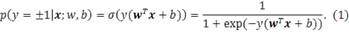A data instance is a vector with each dimension representing a value for some feature. For example, the Iris flower data set we are going to use in this post has 4 features: Sepal Length, Sepal Width, Petal Length and Petal Width. Each instance has a label y indicating the category. The above model only models binary labels. Logistic regression also has multiple categories version, see [Mitchell]. In this post, we focus on the binary version; however multiple versions should be straightforward once we know how to implement the binary one.

The model contains two parameters weight vector w and offset b, which should be estimated/learned on the training data set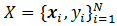. In our derivations below, we will drop the offset b, because we can always add one feature (constant 1) to feature vector x’=[x 1] and hide b into the extended w’=[w b]. Sometimes, a model without the offset parameter could also perform equally well. Because equation (1) measures the probability, we could solve the model parameters by optimizing the joint probability estimated on dataset X.

If we assume that all the instances are independent and identically distributed (i.i.d.). Then the regularized joint probability iswhere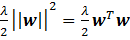is the regularization term for avoiding over-fitting on the training data.

The log version of this likelihood is

We want to find the model parameter w that maximizes this likelihood. P(X) is a concave function with respect to w, so it has a global maximal.

What left is how to optimize this function. BFGS is a Quasi-Newton method that only needs first order gradient while a Newton family method needs second order explicitly. Microsoft Solver Foundation has a limited memory version of BFGS (L-BFGS). (L-BFGS is more popular than BFGS, sometimes people mention BFGS to mean L-BFGS.) There is a memory parameter m in L-BFGS, the space complexity of this algorithm is O(md), where d is the dimension of the variable space. Say, if you want to optimize a function that has 1,000,000 free variables, and set m to 10, then you need only a little more than 1M*10*8/1024^2=76M bytes.

The input for an L-BFGS optimizer is: 1) the function evaluator L(w) and 2) the gradient evaluator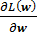: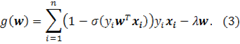The output is the best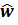that gives the maximum function value.

L-BFGS solver in Microsoft Solver Foundation

Microsoft Solver Foundation already implements an L-BFGS solver. The reader is suggested to read pages 24-27 & 72-75 of MSF-SolverProgrammingPrimer.pdf (on my machine: C:\Program Files\Microsoft Solver Foundation\3.0.1.10599\Documents) for detailed reference. Because the code for optimizing Rosenbrock function is in C#, here I translated it to F#. Note how I translate between delegate type System.Func<> and F# functions.

Btw, I find that using the Microsoft.Solver.Foundatoin.dll in Sho would be more convenient as it packages everything into one DLL.

#r @"C:\Program Files (x86)\Sho 2.0 for .NET 4\packages\Optimizer\Microsoft.Solver.Foundation.dll"
openMicrosoft.SolverFoundation.Common
openMicrosoft.SolverFoundation.Solvers
openMicrosoft.SolverFoundation.Services

letsolverParams = newCompactQuasiNewtonSolverParams()
letsolver = newCompactQuasiNewtonSolver()

//add a row and set it as the goal

letOriginalRosenbrockFunction =
letf (model:INonlinearModel)  (rowVid:int)  (values:ValuesByIndex)  (newValues:bool) =
Math.Pow(1. - values., 2.) + 100. * (Math.Pow(values. - (values. * values.), 2.))
newSystem.Func<INonlinearModel, int, ValuesByIndex, bool, float> (f)

letf (model:INonlinearModel) (rowVid:int) (values:ValuesByIndex) (newValues:bool) (gradient:ValuesByIndex) =
gradient. <- -2. * (1. - values.) - 400. * values. * (values. - (values. * values.))
gradient. <- 200. * (values. - (values. * values.))
newSystem.Action<INonlinearModel, int, ValuesByIndex, bool, ValuesByIndex> (f)

solver.FunctionEvaluator <- OriginalRosenbrockFunction
solver.Solve(solverParams);

Console.WriteLine(solver.ToString());

and the output:

>
Minimize problem
Dimensions = 2
Variable indexes:
1, 2
Starting point = 0, 0
Solution quality is: LocalOptima
Number of iterations performed: 21
Number of evaluation calls: 27
Finishing point =0.999999997671584, 0.999999995285991
Finishing value =5.74844710320033E-18

val it : unit = ()
>

Implementation for logistic regression

We first get a sample data set online:

module Net =
open System.Net

let fetchUrlSimple (url:string) =
let req = WebRequest.Create(url)
let response = req.GetResponse()
use stream = response.GetResponseStream()

type dataset =
{ features: float array array; // (instance = float array) array
mutable labels: int array; //
}

let iris =
let page = Net.fetchUrlSimple @"http://archive.ics.uci.edu/ml/machine-learning-databases/iris/iris.data"
let lines = page.Split([|'\n'|], StringSplitOptions.RemoveEmptyEntries)
let getIrisId (s:string) =
if s = "Iris-setosa" then
2
elif s = "Iris-versicolor" then
-1
else
1

let instances =
lines
|> Array.map (fun line ->
let
s = line.Split ','
let features = [| "1.0"; s.; s.; s.; s. |] |> Array.map float // add a dumy 1.0 into the fatures
let label = getIrisId s.
features, label
)
|> Array.filter (fun (_, label) -> label <> 2)

let F, L = Array.unzip instances
{
features = F;
labels = L;
}

The logistic regression implementation:

module LogReg =
let mutable lambda = 0.1

let dotProduct (x:float array) (g:ValuesByIndex) =
let mutable dot = 0.0
for i=0 to x.Length-1 do
dot <- dot + x.[i] * g.[i+1]
dot

let sigmoid (x:float array) (y:int) (g:ValuesByIndex) =
let mutable dot = 0.0
for i=0 to x.Length-1 do
dot <- dot + x.[i] * g.[i+1]
let z = (float y) * dot
(* code to deal with extream numbers, but with some issues with l(w) calculation
if z > 30.0 then
1.0
elif z < -30.0 then
0.0
else
*)
1.0 / (1.0 + exp (- z))

let sigmoid2 (x:float array) (y:int) (g:float array) =
let mutable dot = 0.0
for i=0 to x.Length-1 do
dot <- dot + x.[i] * g.[i]
1.0 / (1.0 + exp (- (float y) * dot))

let logregValue(ds:dataset) =
let dim = ds.features..Length

let f (model:INonlinearModel) (rowVid:int) (values:ValuesByIndex) (newValues:bool) =
let mutable L = 0.0

for i=1 to dim do
L <- L + values.[i]*values.[i]
L <- - (L * lambda / 2.0)

for i=0 to ds.features.Length-1 do
//L <- L - log (1.0 + exp (- (float ds.labels.[i]) * dotProduct ds.features.[i] values ))
L <- L + log (sigmoid ds.features.[i] ds.labels.[i] values)

// printfn "L = %.10f" L
L

new System.Func<INonlinearModel, int, ValuesByIndex, bool, float> (f)

let dim = ds.features..Length
let f (model:INonlinearModel) (rowVid:int) (values:ValuesByIndex) (newValues:bool) (gradient:ValuesByIndex) =
for j=1 to dim do

for i=0 to ds.features.Length-1 do
let
coef = (1.0 - sigmoid ds.features.[i] ds.labels.[i] values) * (float ds.labels.[i])
for j=1 to dim do

new System.Action<INonlinearModel, int, ValuesByIndex, bool, ValuesByIndex> (f)

let makeSolver(ds:dataset) =

// set the solver parameters
let solverParams = new CompactQuasiNewtonSolverParams()
//solverParams.IterationLimit <- 11
//solverParams.Tolerance <- 1e-4
// the memory parameter m, default 17
solverParams.IterationsToRemember <- 10

// solver
let solver = new CompactQuasiNewtonSolver()

let dim = ds.features..Length
for i=1 to dim do

// add a row and set it as the goal

// set funcation evaluator and gradient evaluator
solver.FunctionEvaluator <- logregValue(ds)

// solve!
solver.Solve(solverParams) |> ignore

// get the optimized point (w) in the solver
let w = Array.create dim 0.0
for i=1 to dim do
w.[i-1] <- solver.GetValue(i).ToDouble()
w

Try our logistic regression model on the Iris dataset:

let sol = LogReg.makeSolver(iris)
let nWrongs = ref 0
for i=0 to iris.features.Length-1 do
let
prob = LogReg.sigmoid2 iris.features.[i] iris.labels.[i] sol
printfn "L = %A sig value = %A label = %A" iris.labels.[i] prob (if prob > 0.5 then "correct" else incr nWrongs; "wrong")

Reference

Tom Mitchell, Generative and Discriminative Classifiers: Naive Bayes and Logistic Regression, new book chapter. This chapter gives a good introduction to logistic regression. For a more theoretic comparison, see: Ng & Jordan: on discriminative vs generative classifiers a comparison of logistic regression and naïve bayes.

Thomas P. Minka, A comparison of numerical optimizers for logistic regression.

It is well written, the first page ready gives a concise introduction to logistic regression. The rest of the paper has a lot of discussion on optimization methods. Tom also has a Matlab package.

Galen Andrew and Jianfeng Gao. Scalable training of L1-regularized log-linear models. In Proceedings of the 24th International Conference on Machine Learning (ICML 2007), pp. 33-40, 2007.

L1-regularized logistic regression with an implementation in C++.

libLBFGS(LBFGS in C): http://www.chokkan.org/software/liblbfgs/

Note

I quite like this post because it combines so many good things together: F#, logistic regression, optimization and playing with sample datasets.

Thursday, February 10, 2011

Introducing Sho and using its libraries from F#

My friend passed me the link for Sho, a data processing and number crunching tool on .Net platform. It is developed in Microsoft Research. I am quite amazed by this tool and like to share a post here.

The frontend of Sho is IronPython. Besides all the standard Python core language and its libraries, Sho also integrates with part of Intel MKL library, which supports linear algebra operations.

There are rumors that there will be a machine learning/data mining library based on Sho in the future.

Key features of Sho

1) IronPython, and its console has some IntelliSense

2) libraries:

* Matrix and linear algebra (Intel MKL©)

both 32bit & 64bit support + multi-threaded

* Statistics (Sho Library)

* Signal processing (Sho Library)

* Optimization (Microsoft Solver Foundation)

3) Interactive visualization

The official site of Sho has some examples and good documents including a reference book.

IronPython?

The language and the open nature make Python a very suitable language for scientific computing. I know that a lot of researchers in data mining area use or occasionally use Python for their research. Three friends of mine are using Python for their research. They are also very good at Matlab. One said to me that he writes his development speed of Python(Scipy/Numpy) and Matlab are about the same. For the running speed, Scipy uses ATLAS + Lapcak, which is about the same as Matlab.

But I think the real power of Python is that your prototype is your deployment. If you ecosystem uses .Net, your number crunching module in IronPython could be ready be used in the system. While for Matlab, deployment is not that easy.

I think IronPython + Sho would be as good as Python+SciPy/Numpy.

I personally don’t quite favor dynamic languages. It is the libraries Sho uses make Sho excellent, F# + Sho libraries rocks too.

Using the Sho API in F#

The following is a sample F# script showing how to use Sho library in F#.

// set the env variable SHODIR to the root of Sho's installation folder
System.Environment.SetEnvironmentVariable("SHODIR", @"C:\Program Files (x86)\Sho 2.0 for .NET 4")

#r @"C:\Program Files (x86)\Sho 2.0 for .NET 4\bin\ShoArray.dll"
#r @"C:\Program Files (x86)\Sho 2.0 for .NET 4\bin\MatrixInterf.dll"

#time

open
ShoNS.Array
open System

let rnd = new Random(1)

let vec = new DoubleArray(3)
vec.FillRandom(rnd)

let arr2 = new DoubleArray(3,3)
arr2.FillRandom(rnd)

// big matrix multiplication
let bigArr = new DoubleArray(1200,20000)
bigArr.FillRandom(rnd)
let bigArr2 = bigArr.Transpose()
let bigArrMulti = bigArr * bigArr2
// Real: 00:00:01.745, CPU: 00:00:10.389, GC gen0: 0, gen1: 0, gen2: 0

// svd
let svd = new SVD(arr2)
printfn "U = %A\nD = %A\nV = %A" svd.D svd.U svd.V

// lu
let lu = new LU(arr2)
printfn "L = %A\nU = %A" lu.L lu.U

Notice that Sho linear algebra library is actually licensed from Intel MKL, which is multi-threaded! (Matlab also uses Intel MKL as its basic linear algebra library.)

Basic operations like matrix multiplication is very fast if it uses more cores, as illustrated above.

Note

Fsi.exe runs in 32bit mode. Thus your cannot create large matrices and other objects in the F# interactive. There are workarounds here.

Tuesday, February 1, 2011

How to quickly learn F#

I haven’t blogged about F# for sometime now because I am busy with my research work recently. But I nearly use F# everyday to conduct experiments. Also I attracted several of my teammates to get interested in F#, so I write the following guide for my teammates to quickly learn F#.

There are other “learning outline” pages such as the wiki page on Stackoverflow. However the following list provides a path for learning F# and hope to reduce the learning time to be as short as possible.

BTW, it is the Chinese New Year now. Wish everyone a warm Spring Festival!

Step 1

Before reading books/articles, watch the three videos by Luca Bolognese (former F# PM), Luke Hoban (F# PM) and Don Syme (creator of F#).

Step 2

Read the book by Chris Smith (former F# team member, now at Google): Programming F#. After reading the book, quickly scan the following two online books:

· F# Wikibook: http://en.wikibooks.org/wiki/Programming:F_Sharp

· The F# Survival Guide: http://www.ctocorner.com/fsharp/book/default.aspx

They basically cover the same content as Programming F#; reading them gives you a refresh of basic F#.

Step 3

Try to solve the first 50 problems at Project Euler: http://projecteuler.net/ and read the solutions at: http://fsharp-euler.wikispaces.com/ if you have difficulty. You can also search the solutions online; there are many blogs on how to solve Project Euler problems using F#. But before reading the solution, try your best and you can learn F# basics very quickly.

Step 3 alternative

You can skip step 3 (or part of it) if you have experience in building a compiler or interpreter and you are interested in programming languages. The ProgramAsData folder contains the free online book, homeworks (some F# template code is provided) and well-edited slides in the Program as Data course by Prof. Peter Sestoft.

Step 4

Read some chapters of Expert F# 2.0 by Don Syme, and use the book as a reference when you program a bigger/real-world project in F#.

[Optional] Read books like CLR via C# to know more about the .Net platform.